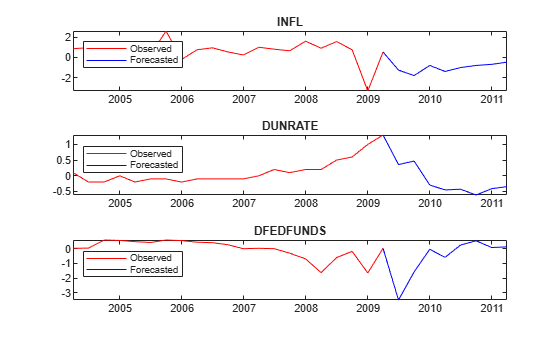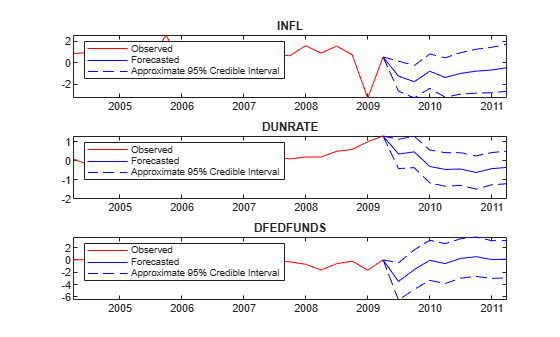# forecast

Forecast responses from Bayesian vector autoregression (VAR) model

## Syntax

``YF = forecast(PriorMdl,numperiods,Y)``
``[YF,YFStd] = forecast(PriorMdl,numperiods,Y)``

## Description

`forecast` is well suited for computing out-of-sample unconditional forecasts of a Bayesian VAR(p) model that does not contain an exogenous regression component. For advanced applications, such as out-of-sample conditional forecasting, VARX(p) model forecasting, missing value imputation, and Gibbs sampler specification for posterior predictive distribution estimation, see `simsmooth`.

example

````YF = forecast(PriorMdl,numperiods,Y)` returns a path of forecasted responses `YF` over the length `numperiods` forecast horizon. Each period in `YF` is the mean of the posterior predictive distribution, which is derived from the posterior distribution of the prior Bayesian VAR(p) model `PriorMdl` given the response data `Y`. The output `YF` represents the continuation of `Y`. `NaN`s in the data indicate missing values, which `forecast` removes using list-wise deletion.```

example

``[YF,YFStd] = forecast(PriorMdl,numperiods,Y)` also returns the corresponding standard deviations of the posterior predictive distribution `YFStd`.`

## Examples

collapse all

Consider the 3-D VAR(4) model for the US inflation (`INFL`), unemployment (`UNRATE`), and federal funds (`FEDFUNDS`) rates.

`$\left[\begin{array}{l}{\text{INFL}}_{t}\\ {\text{UNRATE}}_{t}\\ {\text{FEDFUNDS}}_{t}\end{array}\right]=c+\sum _{j=1}^{4}{\Phi }_{j}\left[\begin{array}{l}{\text{INFL}}_{t-j}\\ {\text{UNRATE}}_{t-j}\\ {\text{FEDFUNDS}}_{t-j}\end{array}\right]+\left[\begin{array}{c}{\epsilon }_{1,t}\\ {\epsilon }_{2,t}\\ {\epsilon }_{3,t}\end{array}\right].$`

For all $t$, ${\epsilon }_{t}$ is a series of independent 3-D normal innovations with a mean of 0 and covariance $\Sigma$. Assume a diffuse prior distribution for the parameters $\left({\left[{\Phi }_{1},...,{\Phi }_{4},\mathit{c}\right]}^{\prime },\Sigma \right)$.

Load the US macroeconomic data set. Compute the inflation rate, stabilize the unemployment and federal funds rates, and remove missing values.

```load Data_USEconModel seriesnames = ["INFL" "UNRATE" "FEDFUNDS"]; DataTimeTable.INFL = 100*[NaN; price2ret(DataTimeTable.CPIAUCSL)]; DataTimeTable.DUNRATE = [NaN; diff(DataTimeTable.UNRATE)]; DataTimeTable.DFEDFUNDS = [NaN; diff(DataTimeTable.FEDFUNDS)]; seriesnames(2:3) = "D" + seriesnames(2:3); rmDataTimeTable = rmmissing(DataTimeTable);```

Create Prior Model

Create a diffuse prior model. Specify the response series names.

```numseries = numel(seriesnames); numlags = 4; PriorMdl = bayesvarm(numseries,numlags,'SeriesNames',seriesnames);```

Forecast Responses

Directly forecast two years (eight quarters) of observations from the posterior predictive distribution. `forecast` estimates the posterior distribution of the parameters, and then forms the posterior predictive distribution.

```numperiods = 8; YF = forecast(PriorMdl,numperiods,rmDataTimeTable{:,seriesnames});```

`YF` is an 8-by-3 matrix of forecasted responses.

Plot the forecasted responses.

```fh = rmDataTimeTable.Time(end) + calquarters(0:8); tiledlayout(3,1) for j = 1:PriorMdl.NumSeries nexttile plot(rmDataTimeTable.Time(end - 20:end),rmDataTimeTable{end - 20:end,seriesnames(j)},'r',... fh,[rmDataTimeTable{end,seriesnames(j)}; YF(:,j)],'b'); legend("Observed","Forecasted",'Location','NorthWest') title(seriesnames(j)) end```Consider the 3-D VAR(4) model of Forecast Responses from Posterior Predictive Distribution.

Load the US macroeconomic data set. Compute the inflation rate, stabilize the unemployment and federal funds rates, and remove missing values.

```load Data_USEconModel seriesnames = ["INFL" "UNRATE" "FEDFUNDS"]; DataTimeTable.INFL = 100*[NaN; price2ret(DataTimeTable.CPIAUCSL)]; DataTimeTable.DUNRATE = [NaN; diff(DataTimeTable.UNRATE)]; DataTimeTable.DFEDFUNDS = [NaN; diff(DataTimeTable.FEDFUNDS)]; seriesnames(2:3) = "D" + seriesnames(2:3); rmDataTimeTable = rmmissing(DataTimeTable);```

Create a diffuse prior model. Specify the response series names.

```numseries = numel(seriesnames); numlags = 4; PriorMdl = bayesvarm(numseries,numlags,'SeriesNames',seriesnames);```

Directly forecast two years (eight quarters) of response observations from the posterior predictive distribution. Return the posterior standard deviations.

```numperiods = 8; [YF,YStd] = forecast(PriorMdl,numperiods,rmDataTimeTable{:,seriesnames});```

`YF` and `YStd` are 8-by-3 matrices of forecasted responses and corresponding standard deviations, respectively.

Plot the forecasted responses and approximate 95% credible intervals.

```fh = rmDataTimeTable.Time(end) + calquarters(0:8); for j = 1:PriorMdl.NumSeries subplot(3,1,j) plot(rmDataTimeTable.Time(end - 20:end),rmDataTimeTable{end - 20:end,seriesnames(j)},'r',... fh,[rmDataTimeTable{end,seriesnames(j)}; YF(:,j)],'b',... fh,[rmDataTimeTable{end,seriesnames(j)}; YF(:,j) + 1.96*YStd(:,j)],'b--',... fh,[rmDataTimeTable{end,seriesnames(j)}; YF(:,j) - 1.96*YStd(:,j)],'b--'); legend("Observed","Forecasted","Approximate 95% Credible Interval",'Location','NorthWest') title(seriesnames(j)) end```## Input Arguments

collapse all

Prior Bayesian VAR model, specified as a model object in this table.

Model ObjectDescription
`conjugatebvarm`Dependent, matrix-normal-inverse-Wishart conjugate model returned by `bayesvarm`, `conjugatebvarm`, or `estimate`
`semiconjugatebvarm`Independent, normal-inverse-Wishart semiconjugate prior model returned by `bayesvarm` or `semiconjugatebvarm`
`diffusebvarm`Diffuse prior model returned by `bayesvarm` or `diffusebvarm`
`normalbvarm`Normal conjugate model with a fixed innovations covariance matrix, returned by `bayesvarm`, `normalbvarm`, or `estimate`

Forecast horizon, or the number of time points in the forecast period, specified as a positive integer.

Data Types: `double`

Presample and estimation sample multivariate response series, specified as a (`numlags + numobs`)-by-`numseries` numeric matrix.

Rows correspond to observations, and the last row contains the latest observation. `forecast` uses the first `numlags` = `PriorMdl.P` observations as a presample to initialize the prior model `PriorMdl` for posterior estimation. `forecast` estimates the posterior using the remaining `numobs` observations and `PriorMdl`.

`numseries` is the number of response variables `PriorMdl.NumSeries`. Columns correspond to individual response variables `PriorMdl.SeriesNames`.

For more details, see Algorithms.

Data Types: `double`

## Output Arguments

collapse all

Path of multivariate response series forecasts, returned as a `numperiods`-by-`numseries` numeric matrix. `YF` is the mean of the posterior predictive distribution of each period in the forecast horizon.

`YF` represents the continuation of the response series `Y`. Rows correspond to observations; row `j` is the `j`-period-ahead forecast. Columns correspond to the columns in `Y`.

Forecast standard deviations, returned as a `numperiods`-by-`numseries` numeric matrix. `YFStd` is the standard deviation of the posterior predictive distribution of each period in the forecast horizon. Dimensions correspond to the dimensions of `YF`.

collapse all

### Bayesian Vector Autoregression (VAR) Model

A Bayesian VAR model treats all coefficients and the innovations covariance matrix as random variables in the m-dimensional, stationary VARX(p) model. The model has one of the three forms described in this table.

ModelEquation
Reduced-form VAR(p) in difference-equation notation
`${y}_{t}={\Phi }_{1}{y}_{t-1}+...+{\Phi }_{p}{y}_{t-p}+c+\delta t+Β{x}_{t}+{\epsilon }_{t}.$`
Multivariate regression
`${y}_{t}={Z}_{t}\lambda +{\epsilon }_{t}.$`
Matrix regression
`${y}_{t}={\Lambda }^{\prime }{z}_{t}^{\prime }+{\epsilon }_{t}.$`

For each time t = 1,...,T:

• yt is the m-dimensional observed response vector, where m = `numseries`.

• Φ1,…,Φp are the m-by-m AR coefficient matrices of lags 1 through p, where p = `numlags`.

• c is the m-by-1 vector of model constants if `IncludeConstant` is `true`.

• δ is the m-by-1 vector of linear time trend coefficients if `IncludeTrend` is `true`.

• Β is the m-by-r matrix of regression coefficients of the r-by-1 vector of observed exogenous predictors xt, where r = `NumPredictors`. All predictor variables appear in each equation.

• ${z}_{t}=\left[\begin{array}{ccccccc}{y}_{t-1}^{\prime }& {y}_{t-2}^{\prime }& \cdots & {y}_{t-p}^{\prime }& 1& t& {x}_{t}^{\prime }\end{array}\right],$ which is a 1-by-(mp + r + 2) vector, and Zt is the m-by-m(mp + r + 2) block diagonal matrix

`$\left[\begin{array}{cccc}{z}_{t}& {0}_{z}& \cdots & {0}_{z}\\ {0}_{z}& {z}_{t}& \cdots & {0}_{z}\\ ⋮& ⋮& \ddots & ⋮\\ {0}_{z}& {0}_{z}& {0}_{z}& {z}_{t}\end{array}\right],$`

where 0z is a 1-by-(mp + r + 2) vector of zeros.

• $\Lambda ={\left[\begin{array}{ccccccc}{\Phi }_{1}& {\Phi }_{2}& \cdots & {\Phi }_{p}& c& \delta & Β\end{array}\right]}^{\prime }$, which is an (mp + r + 2)-by-m random matrix of the coefficients, and the m(mp + r + 2)-by-1 vector λ = vec(Λ).

• εt is an m-by-1 vector of random, serially uncorrelated, multivariate normal innovations with the zero vector for the mean and the m-by-m matrix Σ for the covariance. This assumption implies that the data likelihood is

`$\ell \left(\Lambda ,\Sigma |y,x\right)=\prod _{t=1}^{T}f\left({y}_{t};\Lambda ,\Sigma ,{z}_{t}\right),$`

where f is the m-dimensional multivariate normal density with mean ztΛ and covariance Σ, evaluated at yt.

Before considering the data, you impose a joint prior distribution assumption on (Λ,Σ), which is governed by the distribution π(Λ,Σ). In a Bayesian analysis, the distribution of the parameters is updated with information about the parameters obtained from the data likelihood. The result is the joint posterior distribution π(Λ,Σ|Y,X,Y0), where:

• Y is a T-by-m matrix containing the entire response series {yt}, t = 1,…,T.

• X is a T-by-m matrix containing the entire exogenous series {xt}, t = 1,…,T.

• Y0 is a p-by-m matrix of presample data used to initialize the VAR model for estimation.

### Posterior Predictive Distribution

A posterior predictive distribution of a posterior Bayesian VAR(p) model π(Yf|Y,X) is the distribution of the next τ future response variables after the final observation in the estimation sample Yf = [yT+1, yT+2,…,yT+τ] given the following, marginalized over Λ and Σ:

• Presample and estimation sample response data Y

• Coefficients Λ

• Innovations covariance matrix Σ

• Estimation and future sample exogenous data X

Symbolically,

`$\pi \left({Y}_{f}|Y,X\right)=\int \pi \left({Y}_{f}|Y,X,\Lambda ,\Sigma \right)\pi \left(\Lambda ,\Sigma |Y,X\right)d\Lambda d\Sigma .$`

## Tips

• Monte Carlo simulation is subject to variation. If `forecast` uses Monte Carlo simulation, then estimates and inferences might vary when you call `forecast` multiple times under seemingly equivalent conditions. To reproduce estimation results, set a random number seed by using `rng` before calling `forecast`.

## Algorithms

• If the posterior predictive distribution is analytically intractable (true for most cases), `forecast` implements Markov Chain Monte Carlo (MCMC) sampling with Bayesian data augmentation to compute the mean and standard deviation of the posterior predictive distribution. To do so, `forecast` calls `simsmooth`, which uses a computationally intensive procedure.

• Most Econometrics Toolbox™ `forecast` functions accept an estimated or posterior model object from which to generate forecasts. Such a model encompasses the parametric structure and data. However, the `forecast` function of Bayesian VAR models requires presample and estimation sample data to do the following:

• Perform Bayesian parameter updating to estimate posterior distributions. `forecast` implements MCMC sampling with Bayesian data augmentation, which includes a Kalman filter smoothing step that requires the entire observed series.

• Predict future responses in the presence of two sources of uncertainty:

• Estimation noise ε1,…,εT, which induces parameter uncertainty

• Forecast period noise εT+1,…,εT+`numperiods`

 Litterman, Robert B. "Forecasting with Bayesian Vector Autoregressions: Five Years of Experience." Journal of Business and Economic Statistics 4, no. 1 (January 1986): 25–38. https://doi.org/10.2307/1391384.